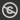Science, Maths & Technology
Free course

# Metric spaces and continuity## Course reviews

This free course contains an introduction to metric spaces and continuity. The key idea is to use three particular properties of the Euclidean distance as the basis for defining what is meant by a general distance function, a metric. Section 1 introduces the idea of a metric space and shows how this concept allows us to generalise the notion of continuity. Section 2 develops the idea of sequences and convergence in metric spaces. Section 3 builds on the ideas from the first two sections to formulate a definition of continuity for functions between metric spaces.

## Course learning outcomes

After studying this course, you should be able to:

• understand the Euclidean distance function on Rn and appreciate its properties, and state and use the Triangle and Reverse Triangle Inequalities for the Euclidean distance function on Rn
• explain the definition of continuity for functions from Rn to Rm and determine whether a given function from Rn to Rm is continuous
• explain the geometric meaning of each of the metric space properties (M1) – (M3) and be able to verify whether a given distance function is a metric
• distinguish between open and closed balls in a metric space and be able to determine them for given metric spaces
• define convergence for sequences in a metric space and determine whether a given sequence in a metric space converges
• state the definition of continuity of a function between two metric spaces.

First Published: 09/03/2018

Updated: 23/05/2018

Skip Rate and Review